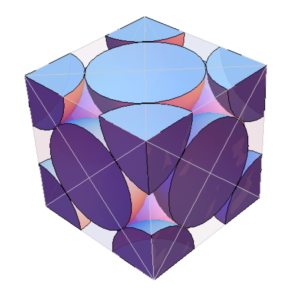## The course

This page describes my notes for the winter 2013 session of the University of Toronto Basic Statistical Mechanics course (PHY452H1S), taught by Prof. Arun Paramekanti, which I took as a non-degree student.

The official course description at the time was:

Classical and quantum statistical mechanics of noninteracting systems; the statistical basis of thermodynamics; ensembles, partition function; thermodynamic equilibrium; stability and fluctuations; formulation of quantum statistics; theory of simple gases; ideal Bose and Fermi systems.

Mathematica notebooks associated with the class are also available.

## The notes.

My notes (399 pages, 6×9″) for this class are available in a few forms:

In all cases, these notes are essentially free (I took about \$0.70 USD royalty for the paper version), so if you feel undercharged, please send me some bitcoin.

## Contributing.

Should you wish to actively contribute typo fixes (or additions, editing, …) to these notes, you can do so by contacting me, or by forking your own copy of the associated git repositories and building the book pdf from source, and submitting a subsequent merge request.

```git clone git@github.com:peeterjoot/latex-notes-compilations.git peeterjoot
cd peeterjoot

submods="figures/phy452-basicstatmech phy452-basicstatmech mathematica latex"
for i in \$submods ; do
git submodule update --init \$i
(cd \$i && git checkout master)
done

export PATH=`pwd`/latex/bin:\$PATH

cd phy452-basicstatmech
make all
```

I reserve the right to impose dictatorial control over any editing and content decisions, and may not accept merge requests as-is, or at all. That said, I’ll probably not refuse reasonable suggestions or merge requests.

## Contents:

• Document Version
• Dedication
• Preface
• Contents
• List of Figures
• 1 What is statististical mechanics and equilibrium
• 1.1 Equilibrium
• 1.2 Probabilities
• 2 Probability
• 2.1 Probability
• 2.2 Central limit theorem
• 2.3 Binomial distribution
• 2.4 Stirling formula
• 2.5 Stirling log approximation
• 2.6 Problems
• 3 Random walk, diffusion, and Maxwell distribution
• 3.1 Spatial Random walk
• 3.2 Continuity equation and Ficks law
• 3.3 Random walk in velocity space
• 3.4 Review: Lead up to Maxwell distribution for gases
• 3.5 What went wrong?
• 3.7 Problems
• 4 Phase space
• 4.1 Phase space
• 4.2 Motion in phase space
• 4.3 Liouville’s theorem
• 4.4 Time averages, and Poincar\351 recurrence theorem
• 4.5 Liouville’s theorem questions on density and current
• 4.6 Liouville’s theorem
• 4.7 Ideal gas and SHO phase space volume calculations
• 4.8 Entropy
• 4.10 Hypersphere volume calculation the easy way
• 4.11 Problems
• 5 Thermodynamics
• 5.1 First law of thermodynamics
• 5.2 Adiabatic and other processes
• 5.3 First law
• 5.4 Examples of work
• 5.5 Heat
• 5.7 Reversible processes
• 5.8 Equilibrium
• 5.9 Cyclic state variable verses non-state variables
• 5.10 Irreversible and reversible processes
• 5.11 Problems
• 6 Statistical and thermodynamic connection
• 6.1 Connections between statistical and thermodynamic views
• 6.2 Ideal gas
• 6.3 Non-ideal gas. General classical system
• 6.4 Canonical ensemble
• 6.5 Canonical partition and Helmholtz free energy
• 6.6 Quantum mechanical picture
• 6.7 Interacting spin
• 6.8 Problems
• 7 Grand canonical ensemble
• 7.1 Grand partition function
• 7.2 Fermions and Bosons
• 7.3 Fermi gas thermodynamics
• 7.4 Bosons
• 7.5 Phonon modes
• 7.6 Problems
• Appendixes
• A Pondering a question on conditional probability
• B Outline of midterm I solutions
• C Phase space trajectories for 1D SHO
• D Thermodynamic identities
• E Addition of two one half spins
• F Langevin small approximation
• G Relativisitic generalization of statistical mechanics
• H Relativisitic density of states
• I Velocity volume element to momentum volume element
• J Unresolved question on energy distribution around mean energy
• K Non integral binomial coefficient
• L Fermi function expansion for thermodynamic quantities
• M Bernoulli polynomials and numbers and Euler-MacLauren summation
• N Summary of relations and helpful formulas (cheat sheet fodder)
• O Mathematica notebooks
• Bibliography

## Changelog

phy452.V0.1.11.pdf

• Fix latex errors that caused NO page numbers!

phy452.V0.1.10.pdf

• Re-export mathematica based figures as .pdf instead of .png
• Add commas and periods into equation,dmath contexts.
• remove blank lines between text and equation/dmath that are connected.
• switch to 6×9 format for kindle direct publishing and reformat various equations that overflowed page boundaries.
• use tcolorbox for tables
• suppress page numbers for list of figures, table of contents, and first page in chapters.This is a hack to deal with my hacking of classicthesis.sty, as it wants to put some of those page
numbers in an unprintable region that kdp doesn’t like (and won’t ignore.)
• replace crude battery/resistor diagram with an svg based figure (with transparency)
• shrink various figures.
• periods on chapter, section, and figure captions.
• move versioning to separate file: make.revision